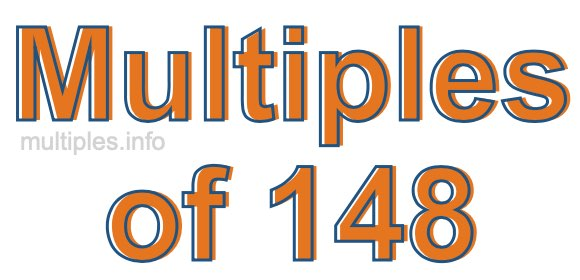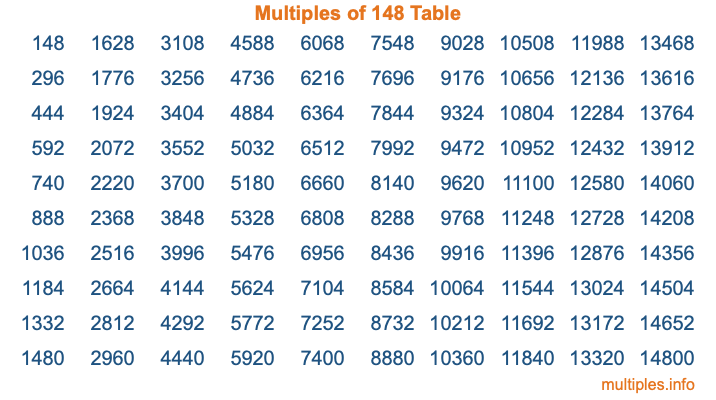Multiples of 148Welcome to the Multiples of 148 page. Here we will first teach you everything you will ever need to know about the multiples of 148, and then give you a study guide summary of everything we taught you to make sure you remember it all. Use this page to look up facts and learn information about the multiples of 148. This page will make you a multiples of one hundred forty-eight expert!

Definition of Multiples of 148
Multiples of 148 are all the numbers that when divided by 148 equal an integer. Each of the multiples of 148 are called a multiple. A multiple of 148 is created by multiplying 148 by an integer.

Therefore, to create a list of multiples of 148, you start with 1 multiplied by 148, then 2 multiplied by 148, then 3 multiplied by 148, and so on for as long as you want. Thus, the list of the first five multiples of 148 is 148, 296, 444, 592, and 740. To see a larger list of multiples of 148, see the printable image of Multiples of 148 further down on this page. We also have a category where you can choose any nth multiple of 148.

Multiples of 148 Checker
The Multiples of 148 Checker below checks to see if any number of your choice is a multiple of 148. In other words, it checks to see if there is any number (integer) that when multiplied by 148 will equal your number. To do that, we divide your number by 148. If the the quotient is an integer, then your number is a multiple of 148.

Is  a multiple of 148?

Least Common Multiple of 148 and ...
A Least Common Multiple (LCM) is the lowest multiple that two or more numbers have in common. This is also called the smallest common multiple or lowest common multiple and is useful to know when you are adding our subtracting fractions. Enter one or more numbers below (148 is already entered) to find the LCM.

Check out our LCM Calculator if you need more details about the Least Common Multiple or if you need the LCM for different numbers for adding and subtraction fractions.

nth Multiple of 148
As we stated above, 148 is the first multiple of 148, 296 is the second multiple of 148, 444 is the third multiple of 148, and so on. Enter a number below to find the nth multiple of 148.

th multiple of 148

Multiples of 148 vs Factors of 148
148 is a multiple of 148 and a factor of 148, but that is where the similarities end. All postive multiples of 148 are 148 or greater than 148. All positive factors of 148 are 148 or less than 148.

Below is the beginning list of multiples of 148 and the factors of 148 so you can compare:

Multiples of 148: 148, 296, 444, 592, 740, etc.

Factors of 148: 1, 2, 4, 37, 74, 148

As you can see, the multiples of 148 are all the numbers that you can divide by 148 to get a whole number. The factors of 148, on the other hand, are all the whole numbers that you can multiply by another whole number to get 148.

It's also interesting to note that if a number (x) is a factor of 148, then 148 will also be a multiple of that number (x).

Multiples of 148 vs Divisors of 148
The divisors of 148 are all the integers that 148 can be divided by evenly. Below is a list of the divisors of 148.

Divisors of 148: 1, 2, 4, 37, 74, 148

The interesting thing to note here is that if you take any multiple of 148 and divide it by a divisor of 148, you will see that the quotient is an integer.

Multiples of 148 Table
Below is an image of the first 100 multiples of 148 in a table. The table is in chronological order, column by column. The first column has the first ten multiples of 148, the second column has the next ten multiples of 148, and so on.The Multiples of 148 Table is also referred to as the 148 Times Table or Times Table of 148. You are welcome to print out our table for your studies.

Negative Multiples of 148
Although not often discussed or needed in math, it is worth mentioning that you can make a list of negative multiples of 148 by multiplying 148 by -1, then by -2, then by -3, and so on, to get the following list of negative multiples of 148:

-148, -296, -444, -592, -740, etc.

Multiples of 148 Summary
Below is a summary of important Multiples of 148 facts that we have discussed on this page. To retain the knowledge on this page, we recommend that you read through the summary and explain to yourself or a study partner why they hold true.

There are an infinite number of multiples of 148.

A multiple of 148 divided by 148 will equal a whole number.

148 divided by a factor of 148 equals a divisor of 148.

The nth multiple of 148 is n times 148.

The largest factor of 148 is equal to the first positive multiple of 148.

148 is a multiple of every factor of 148.

148 is a multiple of 148.

A multiple of 148 divided by a divisor of 148 equals an integer.

148 divided by a divisor of 148 equals a factor of 148.

Any integer times 148 will equal a multiple of 148.

Multiples of a Number
Here you can get the multiples of another number, all with the same attention to detail as we did for multiples of 148 on this page.

Multiples of
Multiples of 149
Did you find our page about multiples of one hundred forty-eight educational? Do you want more knowledge? Check out the multiples of the next number on our list!